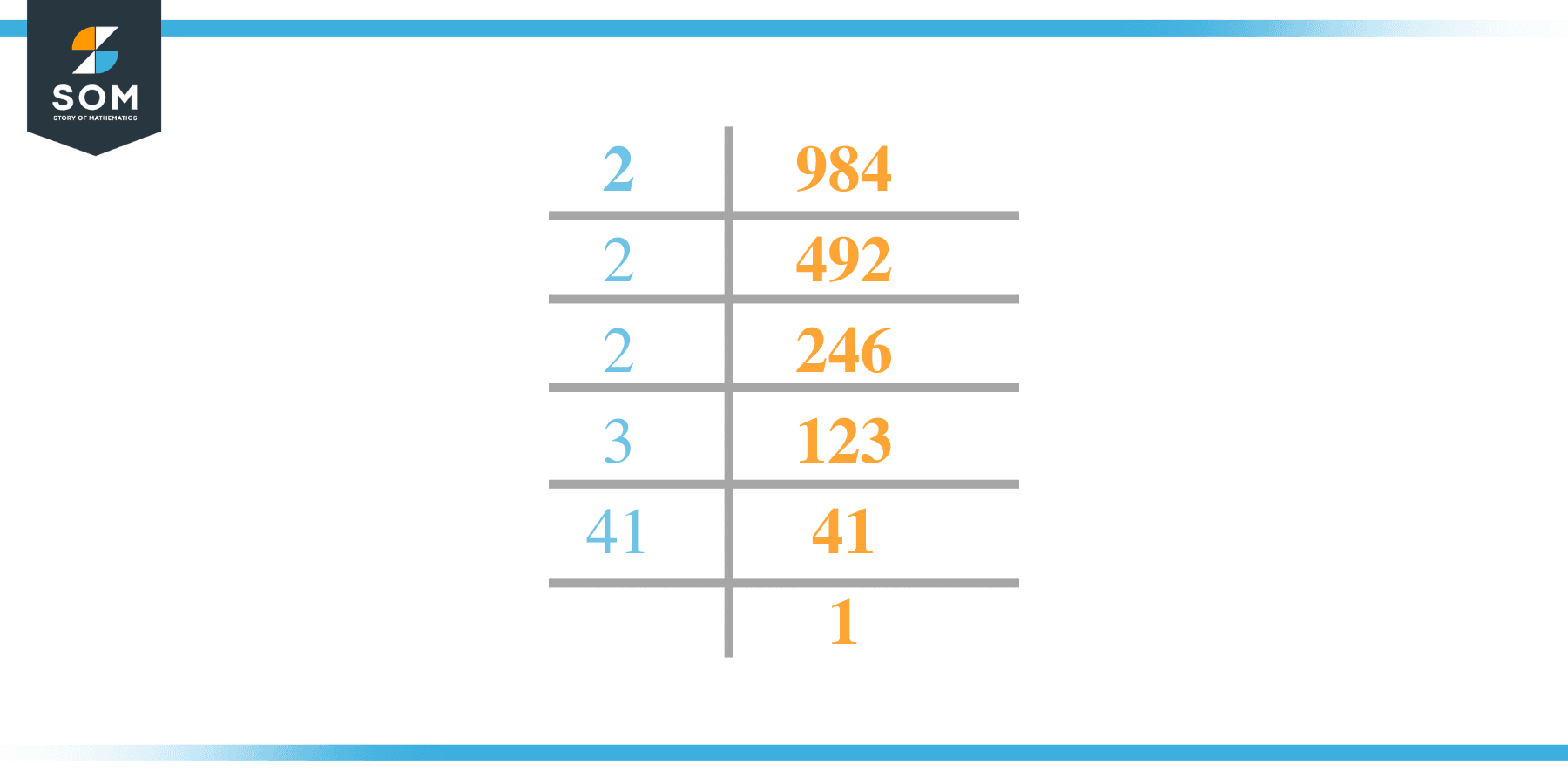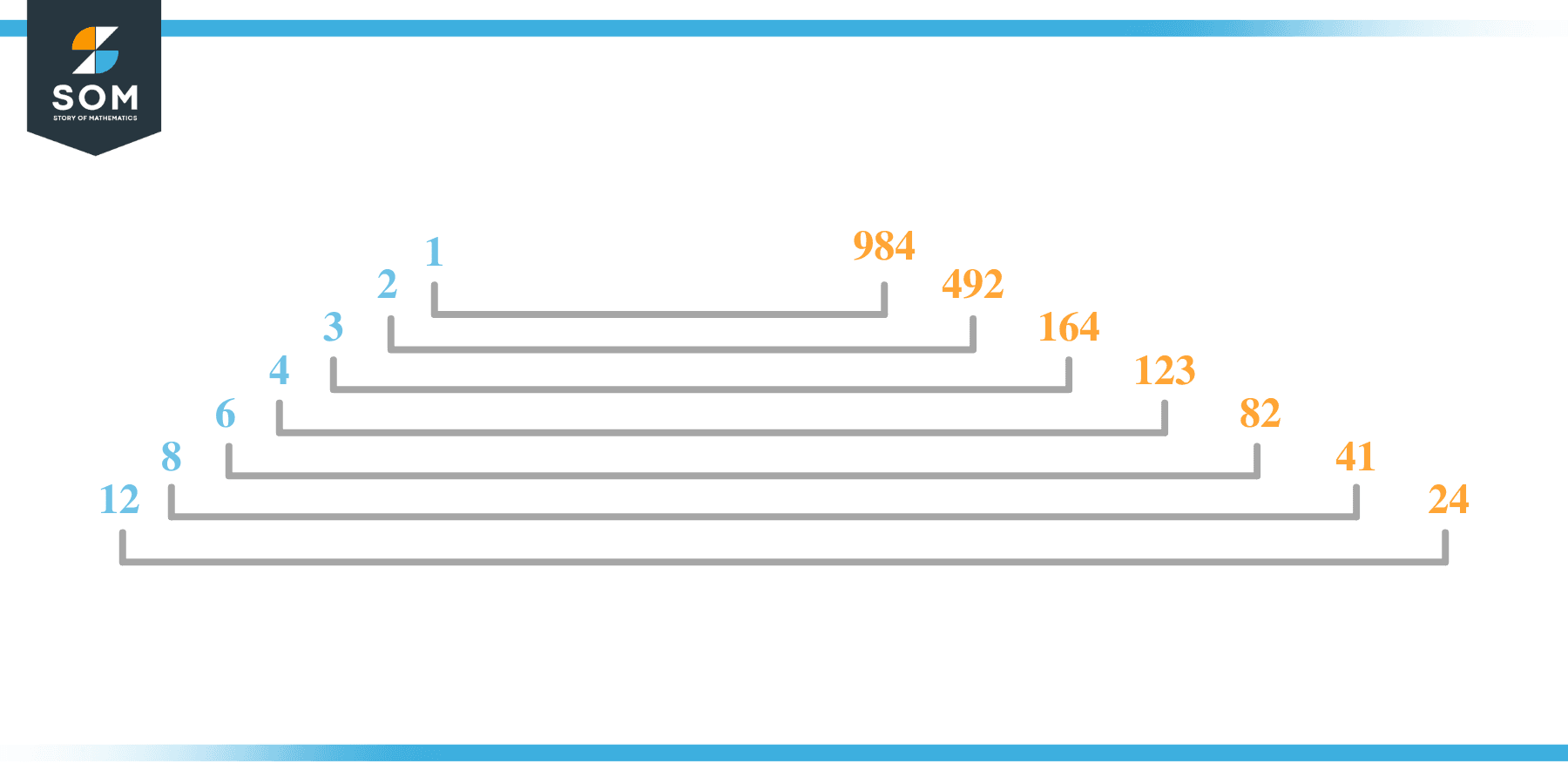# Factors of 984: Prime Factorization, Methods, and Examples

The factors of 984 are the listing of the numbers that are divisible by the number 984. The factors of 984 can be positive or negative. The number 984 is an even number.It is also called a composite number as it has more than two factors.

### Factors of 984

Here are the factors of number 984.

Factors of 984: 1, 2, 3, 4, 6, 8, 12, 24, 41, 82, 123, 164, 246, 328, 492, and 984

### Negative Factors of 984

The negative factors of 984 are similar to their positive aspects, just with a negative sign.

Negative Factors of 984: –1, -2, -3, -4, -6, -8, -12, -24, -41, -82, -123, -164, -246, -328, -492, and -984

### Prime Factorization of 984

The prime factorization of 984 is the way of expressing its prime factors in the product form.

Prime Factorization: 2 x 2 x 2 x 3 x 41

In this article, we will learn about the factors of 984 and how to find them using various techniques such as upside-down division, prime factorization, and factor tree.

## What Are the Factors of 984?

The factors of 984 are 1, 2, 3, 4, 6, 8, 12, 24, 41, 82, 123, 164, 246, 328, 492, and 984. These numbers are the factors as they do not leave any remainder when divided by 984.

The factors of 984 are classified as prime numbers and composite numbers. The prime factors of the number 984 can be determined using the prime factorization technique.

## How To Find the Factors of 984?

You can find the factors of 984 by using the rules of divisibility. The divisibility rule states that any number, when divided by any other natural number, is said to be divisible by the number if the quotient is the whole number and the resulting remainder is zero.

To find the factors of 984, create a list containing the numbers that are exactly divisible by 984 with zero remainders. One important thing to note is that 1 and 984 are 984’s factors, as every natural number has 1 and the number itself as its factor.

1 is also called the universal factor of every number. The factors of 984 are determined as follows:

$\dfrac{984}{1} = 984$

$\dfrac{984}{2} = 492$

$\dfrac{984}{3} = 328$

$\dfrac{984}{4} = 246$

$\dfrac{984}{6} = 164$

$\dfrac{984}{8} = 123$

$\dfrac{984}{12} = 82$

$\dfrac{984}{24} = 41$

Therefore, 1, 2, 3, 4, 6, 8, 12, 24, 41, 82, 123, 164, 246, 328, 492, and 984 are the factors of 984.

### Total Number of Factors of 984

For 984, there are sixteen positive factors and sixteen negative ones. So in total, there are thirty-two factors of 984.

To find the total number of factors of the given number, follow the procedure mentioned below:

1. Find the factorization/prime factorization of the given number.
2. Demonstrate the prime factorization of the number in the form of exponent form.
3. Add 1 to each of the exponents of the prime factor.
4. Now, multiply the resulting exponents together. This obtained product is equivalent to the total number of factors of the given number.

By following this procedure, the total number of factors of 984 is given as:

The factorization of 984 is 1 x 2$^3$ x 3 x 41.

The exponent of 1, 3, and 41 is 1. The exponent of 2 is 3.

Adding 1 to each and multiplying them together results in thirty-two.

Therefore, the total number of factors of 984 is 32. Sixteen are positive, and sixteen factors are negative.

### Important Notes

Here are some essential points that must be considered while finding the factors of any given number:

• The factor of any given number must be a whole number.
• The factors of the number cannot be in the form of decimals or fractions.
• Factors can be positive as well as negative.
• Negative factors are the additive inverse of the positive factors of a given number.
• The factor of a number cannot be greater than that number.
• Every even number has 2 as its prime factor, the smallest prime factor.

## Factors of 984 by Prime FactorizationThe number 984 is a composite. Prime factorization is a valuable technique for finding the number’s prime factors and expressing the number as the product of its prime factors.

Before finding the factors of 984 using prime factorization, let us find out what prime factors are. Prime factors are the factors of any given number that are only divisible by 1 and themselves.

To start the prime factorization of 984, start dividing by its most minor prime factor. First, determine that the given number is either even or odd. If it is an even number, then 2 will be the smallest prime factor.

Continue splitting the quotient obtained until 1 is received as the quotient. The prime factorization of 984 can be expressed as:

984 = 2 x 2 x 2 x 3 x 41

## Factors of 984 in PairsThe factor pairs are the duplet of numbers that, when multiplied together, result in the factorized number. Factor pairs can be more than one depending on the total number of factors given.

For 984, the factor pairs can be found as follows:

1 x 984 = 984

2 x 492 = 984

3 x 164 = 984

4 x 123 = 984

6 x 82 = 984

8 x 41 = 984

12 x 24 = 984

The possible factor pairs of 984 are given as (1, 984), (2, 492), (3, 164), (4, 123), (6, 82), (8, 41), and (12, 24).

All these numbers in pairs, when multiplied, give 984 as the product.

The negative factor pairs of 984 are given as:

-1 x -984 = 984

-2 x -492 = 984

-3 x -164 = 984

-4 x -123 = 984

-6 x -82 = 984

-8 x -41 = 984

-12 x -24 = 984

It is important to note that in negative factor pairs, the minus sign has been multiplied by the minus sign, due to which the resulting product is the original positive number. Therefore, 1, -2, -3, -4, -6, -8, -12, -24, -41, -82, -123, -164, -246, -328, -492, and -984 are called negative factors of 984.

The list of all the factors of 984, including positive as well as negative numbers, is given below.

Factor list of 984: 1, -1, 2, -2, 3, -3, 4, -4, 6, -6, 8, -8, 12, -12, 24, -24, 41, -41, 82, -82, 123, -123, 164, -164, 246, -246, 328, -328, 492, -492, 984, and -984

## Factors of 984 Solved Examples

To better understand the concept of factors, let’s solve some examples.

### Example 1

How many factors of 984 are there?

### Solution

The total number of Factors of 984 is sixteen.

Factors of 984 are 1, 2, 3, 4, 6, 8, 12, 24, 41, 82, 123, 164, 246, 328, 492, and 984.

### Example 2

Find the factors of 984 using prime factorization.

### Solution

The prime factorization of 984 is given as:

984 $\div$ 2 = 492

492 $\div$ 2 = 246

246 $\div$ 2 = 123

123 $\div$ 3 = 41

41 $\div$ 41 = 1

So the prime factorization of 984 can be written as:

2 x 2 x 2 x 3 x 41 = 984